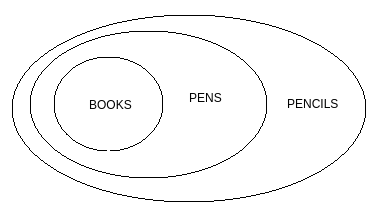# Directions : Given two statements, verify the conclusions and mark the answer as given below. Statements: I.          All books are pens                    II.          All pens are pencils Conclusions: I.          All books are pencils                 II.          Some pens are pencils   Option 1)   if only conclusion I follow.   Option 2)   If only conclusion II follows.   Option 3)   If either conclusion I or II follows.   Option 4)   If neither of the two conclusions follows.   Option 5)   If both conclusions follow